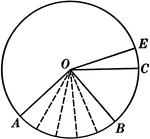### Central Angles and Arcs in a Circle

Illustration of a circle that can be used to show that an "angle at the center of a circle is measured…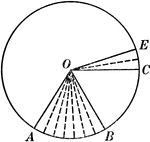### Central Angles and Arcs in a Circle

Illustration of a circle that can be used to show that an "angle at the center of a circle is measured…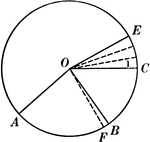### Central Angles and Arcs in a Circle

Illustration of a circle that can be used to show that an "angle at the center of a circle is measured…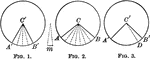### Equal Circles With Intercepted Arcs

Illustration of equal circles to show that two central angles have the same ratio as their intercepted…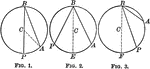### Circles With Inscribed Angles

Illustration of equal circles to show that an inscribed angle is measured by half the arc intercepted…